# The HPLMIXED Procedure

### MODEL Statement

MODEL dependent = <fixed-effects></ options> ;

The MODEL statement names a single dependent variable and the fixed effects, which determine the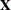matrix of the mixed model. (For details, see the section Specification and Parameterization of Model Effects of Chapter 4: Shared Statistical Concepts.) The MODEL statement is required.

An intercept is included in the fixed-effects model by default. If no fixed effects are specified, only this intercept term is fit. The intercept can be removed by using the NOINT option.

Table 8.3 summarizes options in the MODEL statement. These are subsequently discussed in detail in alphabetical order.

Table 8.3: Summary of Important MODEL Statement Options

Option

Description

Model Building

Excludes the fixed-effect intercept from model

Statistical Computations

ALPHA=Determines the confidence level () for fixed effects

Specifies the method for computing denominator degrees of freedom

Statistical Output

Displays confidence limits for fixed-effects parameter estimates

Displays fixed-effects parameter estimates

You can specify the following options in the MODEL statement after a slash (/).

ALPHA=number

sets the confidence level to be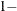number for each confidence interval of the fixed-effects parameters. The value of number must be between 0 and 1; the default is 0.05.

CL

requests that t-type confidence limits be constructed for each of the fixed-effects parameter estimates. The confidence level is 0.95 by default; this can be changed with the ALPHA= option.

DDFM=NONE | RESIDUAL

specifies the method for computing the denominator degrees of freedom for the tests of fixed effects.

The DDFM=RESIDUAL option performs all tests by using the residual degrees of freedom,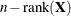, whereis the number of observations used. It is the default degrees-of-freedom method.

DDFM=NONE specifies that no denominator degrees of freedom be applied. PROC HPLMIXED then essentially assumes that infinite degrees of freedom are available in the calculation of
-values. The-values fortests are then identical to-values that are derived from the standard normal distribution. In the case of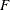tests, the-values equal those of chi-square tests determined as follows: if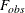is the observed value of thetest withnumerator degrees of freedom, then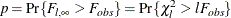NOINT

requests that no intercept be included in the model. (An intercept is included by default.)

SOLUTION
S

requests that a solution for the fixed-effects parameters be produced. Using notation from the section Linear Mixed Models Theory, the fixed-effects parameter estimates are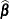and their approximate standard errors are the square roots of the diagonal elements of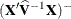.

Along with the estimates and their approximate standard errors, a t statistic is computed as the estimate divided by its standard error. The Pr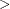|t| column contains the two-tailed-value that corresponds to the t statistic and associated degrees of freedom. You can use the CL option to request confidence intervals for all of the parameters; they are constructed around the estimate by using a radius that is the product of the standard error times a percentage point from the t distribution.